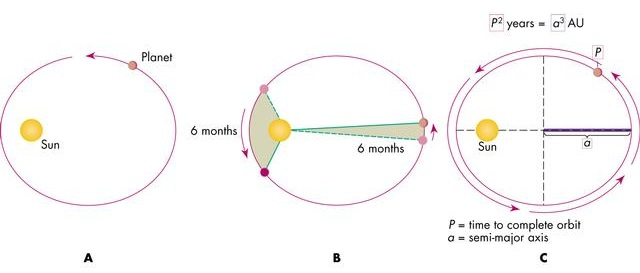# WHAT IS KEPLER’S LAW OF MOTION OR KEPLER’S LAW OF PLANETARY MOTION

#### Q. :Write Kepler’s Law of Motion.Or   Write Kepler’s Law of Planetary Motion.Kepler’s Laws : Kepler stated the three laws of planetary motion as follows :
1. The Law of Elliptical Orbits : Every planet moves in an elliptical orbit around the sun, and the sun being at one of the foci.
2. The Law of Areas : Straight line joining the sum to the planet sweeps equal areas in equal intervats of time.

3. The Harmonic Law : Square of the time period of a planet is directly proportional to the cube of its mean distance from the sun.